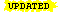#Mathematics

This is our Mathematics page with links to various topics we've written up.

Affiliate disclosure: we get a small commission for purchases made through the above links

### Number theory

Elementary Number Theory
These useful principles of elementary number theory are helpful in understanding the theory behind the RSA algorithm. This includes a list of the first 1000 primes and the the first 10,000 primes.
Number theory: The multiplicative group modulo p
Looks at the multiplicative group modulo p for a prime p which is used in public key cryptography using discrete logarithms. We consider some of the properties relevant to its use in cryptography and recap on some basic group theory.
Number theory: Solving the discrete logarithm problem with bdcalc
Looks at the discrete logarithm problem and how it can be solved (:-) using bdcalc available from the bdcalc page.
Computing a cube root in hexadecimal
Shows how to compute the cube root of a number in hexadecimal format and describes the algorithm to find the exact digits. It includes source code in ANSI C that computes the first 64 bits of the fractional parts of the cube roots of the first eighty prime numbers.
The Euclidean Algorithm and the Extended Euclidean Algorithm
We look at the Euclidean algorithm and how to use it. We solve typical exam questions and show how to do the calculations by hand. We then look at how it can be adapted to find the modular inverse of a number and the extended Euclidean algorithm.
The Chinese Remainder Theorem (CRT) and Gauss's algorithm
We look at the Chinese Remainder Theorem (CRT), Gauss's algorithm to solve simultaneous linear congruences, a simpler method to solve congruences for small moduli, and an application of the theorem to break the RSA algorithm when someone sends the same encrypted message to three different recipients using the same exponent of e=3.
Using the CRT with RSA
We look at how the Chinese Remainder Theorem (CRT) can be used to speed up the calculations for the RSA algorithm. We show how the CRT representation of numbers in Zn can be used to perform modular exponentiation about four times more efficiently using some extra values pre-computed from the prime factors of n, and how Garner's formula is used.
RSA: how to factorize N given d
This page explains how to factorize the RSA modulus N given the public and private exponents, e and d.
Number theory: Dirichlet character table generatorA generator of tables of non-zero values for Dirichlet characters modulo k up to k=62.

### Algorithms

Bloom filters
A look at Bloom filters, a useful algorithm in computer science, and a Bloom filter calculator to find the optimum parameters.

### Linear algebra and coding theory

Linear algebra: Pure Python matrix tools over Zq
Pure Python code to carry out matrix operations (add, multiply, invert, etc) over Zq (i.e. computations are carried out modulo q) where q is an integer greater than 1.
Linear algebra: Transform a matrix to row canonical form
Use this calculator to transform a matrix into row canonical form. This is also called reduced row echelon form (RREF). The theory is explained at Transforming a matrix to reduced row echelon form.
Coding theory: Transform a generator matrix to standard form
This matrix calculator uses the techniques described in Chapters 5 and 7 of Raymond Hill's A First Course in Coding Theory (OUP, 1986) to transform a generator matrix or parity-check matrix of a linear [n,k]-code into standard form. It works over GF(q) for q = 2,3,4,5,7,11.
2D Affine transformations
A really neat diagram showing the effect of applying various 2D affine transformation matrices on a unit square. Image by CMG Lee licensed under (CC BY-SA 3.0).

### Statistics

Statistics: An on-line calculator for the binomial distribution
Calculates a table of the binomial distribution for given parameters and displays graphs of the probability distribution function and cumulative distribution function. Includes background notes on the theory and examples.
Statistics: An on-line calculator for the chi-square distribution
Compute the p-value for a given chi-square statistic, or compute the inverse given the p-value, with the option to display a graph of your results.

### Set theory

Set theory: De Morgan's laws explained in graphical form
This page explains de Morgan's laws, two important results in set theory concerning complementation, using Venn diagrams.

### Classic works

Cauchy's proof of the inequality of arithmetic and geometric means, a rough translation of his original 1821 paper.

### Graph theory

Some notes we prepared for students doing elementary graph theory.